Next: White-Dwarf Stars Up: Quantum Statistics Previous: Conduction Electrons in Metal

# Sommerfeld Expansion

Let us examine the conduction electrons in a metal in slightly more detail. In particular, let us try to obtain a more exact expression for the electronic specific heat. We saw in the previous section that the Fermi energy,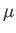, is determined by the equation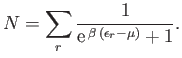(8.154)

Likewise, the mean electron energy is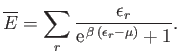(8.155)

Because, in general, the energies of the quantum states are very closely spaced, the sums in the previous two expressions can be replaced by integrals. Now, according to Section 8.12, the number of quantum states per unit volume with wavenumbers in the range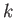to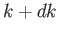is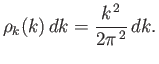(8.156)

However, the energy of a state with wavenumberis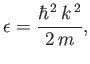(8.157)

where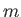is the electron mass. Let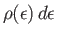be the number of electrons whose energies lies in the range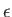to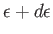. It follows that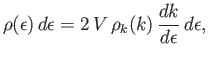(8.158)

where the factor of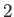is to take into account the two possible spin states which exist for each translational state. Hence,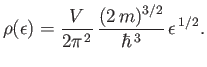(8.159)

Moreover, Equations (8.154) and (8.155) become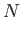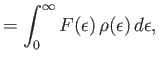(8.160)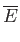(8.161)

where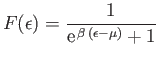(8.162)

is the Fermi function.

The integrals on the right-hand sides of Equations (8.160) and (8.161) are both of the general form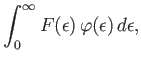(8.163)

where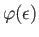is a smoothly varying function of. Let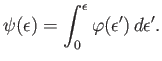(8.164)

We can integrate Equation (8.163) by parts to give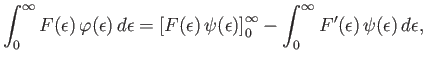(8.165)

which reduces to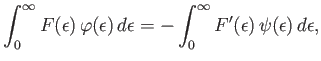(8.166)

because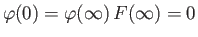. Here,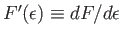.

Now, if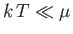then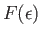is a constant everywhere, apart from a thin region of thickness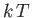, centered on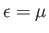. (See Figure 8.4.) It follows that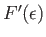is approximately zero everywhere, apart from in this region. Hence, the relatively slowly-varying function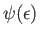can be Taylor expanded about: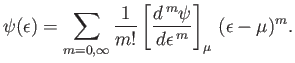(8.167)

Thus, Equation (8.166) becomes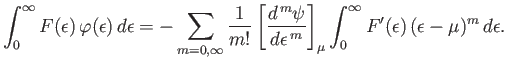(8.168)

From Equation (8.162), we have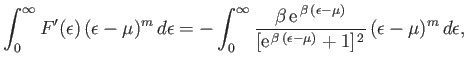(8.169)

which becomes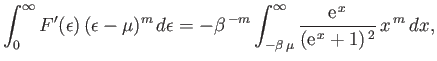(8.170)

where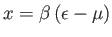. However, because the integrand has a sharp maximum at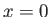, and because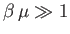, we can replace the lower limit of integration by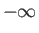with negligible error. Thus, we obtain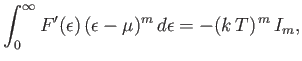(8.171)

where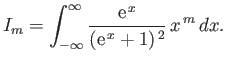(8.172)

Note that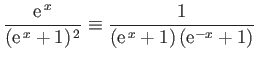(8.173)

is an even function of. It follows, by symmetry, that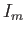is zero whenis odd. Moreover,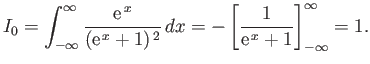(8.174)

Finally, it can be demonstrated that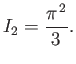(8.175)

(See Exercise 3.) Hence, we deduce that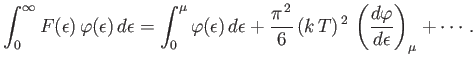(8.176)

This expansion is known as the Sommerfeld expansion, after its inventor, Arnold Sommerfeld.

Equation (8.160) yields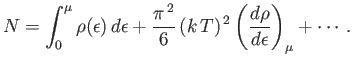(8.177)

However, it follows from Equation (8.159) that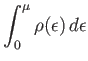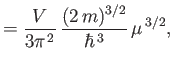(8.178)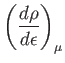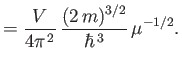(8.179)

Hence,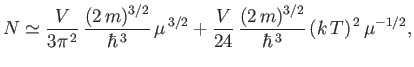(8.180)

which can also be written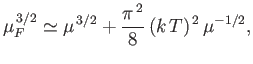(8.181)

where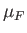is the Fermi energy at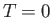. [See Equation (8.144).] The previous equation can be rearranged to give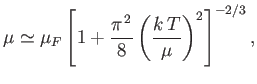(8.182)

which reduces to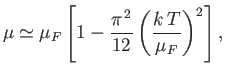(8.183)

assuming that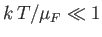. Figure 8.6 shows a comparison between the previous approximate expression for the temperature variation of the Fermi energy of a degenerate electron gas and the numerically-calculated exact value. It can be seen that our approximate expression is surprisingly accurate (at least, for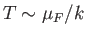).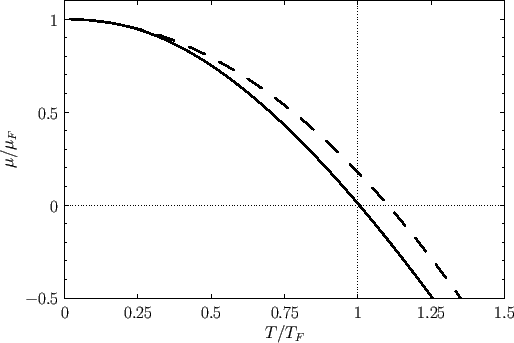Equation (8.161) yields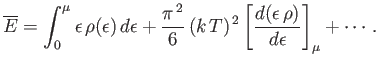(8.184)

However, it follows from Equation (8.159) that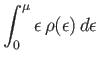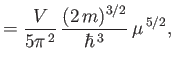(8.185)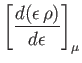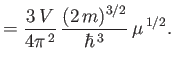(8.186)

Hence,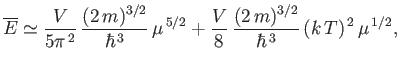(8.187)

which can also be written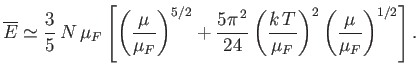(8.188)

Making use of Equation (8.183), and only retaining terms up to second order in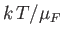, we obtain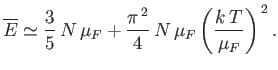(8.189)

Hence, the specific heat capacity of the conduction electrons becomes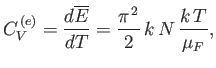(8.190)

and the molar specific heat is written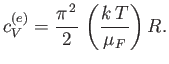(8.191)

Of course, because, this value is much less than the classical estimate,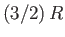.Next: White-Dwarf Stars Up: Quantum Statistics Previous: Conduction Electrons in Metal
Richard Fitzpatrick 2016-01-25